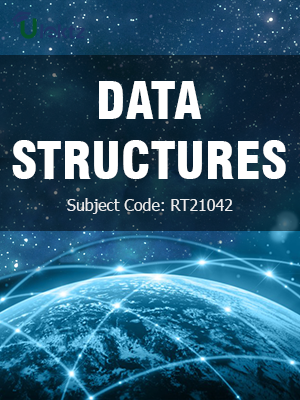uLektz apps

•My WalletMy Order
•My Profile
•My Connections
•My Books
•My Videos
•My Tests
•My Calender
•My Messages
•My Shopping Cart
•My Orders
•Account Settings
•Help

uLektz apps

Book DetailsData Structures

Course Code:RT21042

Author:uLektz

University:

Regulation:2013

Categories:Computer Science

Format :ePUB3 (DRM Protected)

Type :eBook

FREE

Description :Data Structures of RT21042 covers the latest syllabus prescribed by JNTU Kakinada for regulation 2013. Author: uLektz, Published by uLektz Learning Solutions Private Limited.

Note : No printed book. Only ebook. Access eBook using uLektz apps for Android, iOS and Windows Desktop PC.

Topics
UNIT I:INTRODUCTION TO DATA STRUCTURE:

1.1 Data structure- Definition, types of data structures

1.2 Recursion: Definition, Design Methodology and Implementation of recursive algorithms, Linear and binary recursion, recursive algorithms for factorial function

1.3 GCD computation, Fibonacci sequence, Towers of Hanoi, Tail recursion

1.4 List Searches using Linear Search, Binary Search, Fibonacci Search

1.5 Sorting Techniques: Basic concepts, Sorting by: insertion (Insertion sort), selection (heap sort), exchange (bubble sort, quick sort), distribution (radix sort) and merging (merge sort) Algorithms

UNIT II: STACKS AND QUEUES

2.1 Stacks and Queues: Basic Stack Operations, Representation of a Stack using Arrays

2.2 Stack Applications: Reversing list, Factorial Calculation, Infix to postfix Transformation, Evaluating Arithmetic Expressions

2.3 Queues: Basic Queues Operations, Representation of a Queue using array, Implementation of Queue Operations using Stack

2.4 Applications of Queues-Round robin Algorithm, Circular Queues, Priority Queues

UNIT IV: TREES

4.1 Trees: Basic tree concepts, Binary Trees: Properties, Representation of Binary Trees using arrays and linked lists

4.2 Operations on a Binary tree, Binary Tree Traversals (recursive), Creation of binary tree from in, pre and post order traversals

5.1 Advanced concepts of Trees: Tree Travels using stack (non recursive), Threaded Binary Trees

5.2 Binary search tree, Basic concepts, BST operations: insertion, deletion

5.3 Balanced binary trees– need, basics and applications in computer science (No operations)

UNIT VI: GRAPHS

6.1 Graphs: Basic concepts, Representations of Graphs: using Linked list and adjacency matrix, Graph algorithms

6.2 Graph Traversals (BFS & DFS), applications: Dijkstra’s shortest path, Transitive closure

6.3 Minimum Spanning Tree using Prim’s Algorithm

6.4 Warshall’s Algorithm (Algorithmic Concepts Only, No Programs required)

Related Books